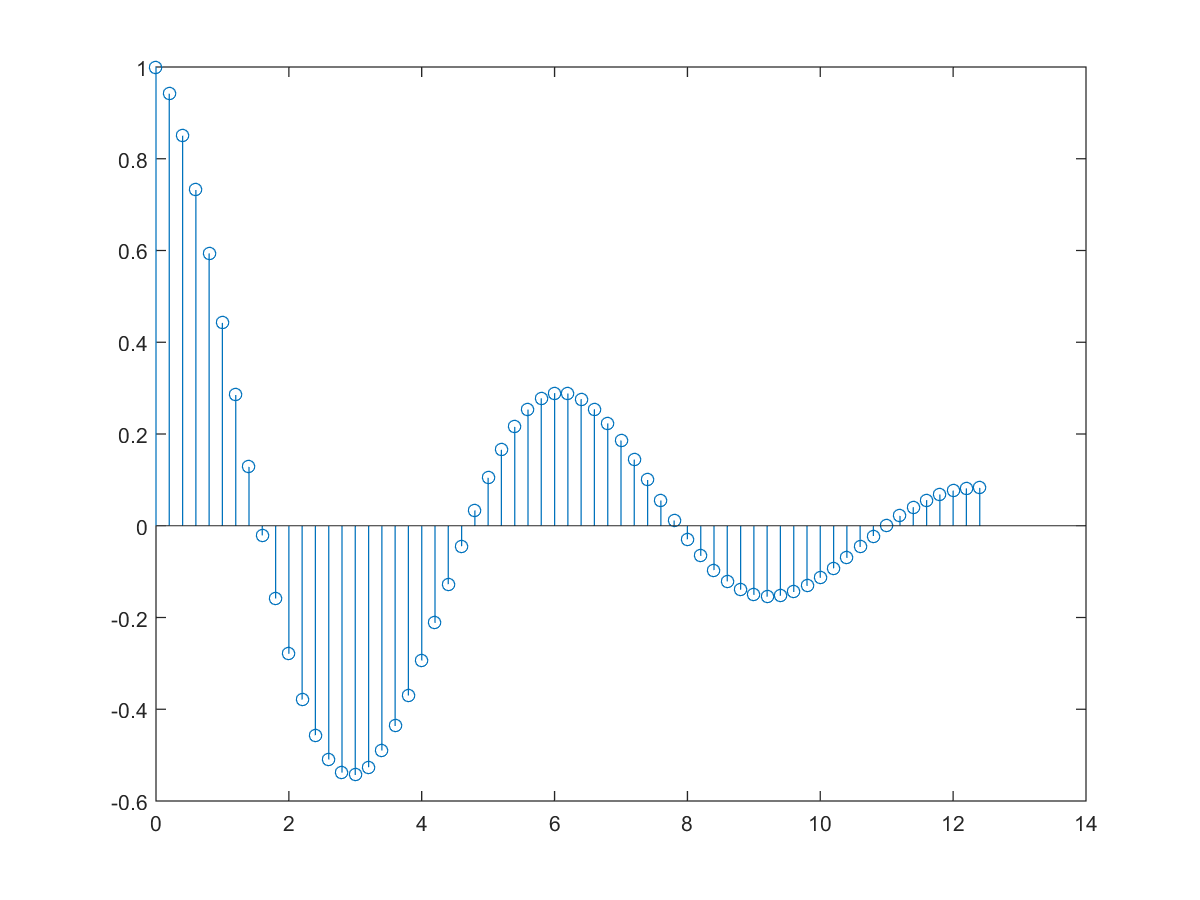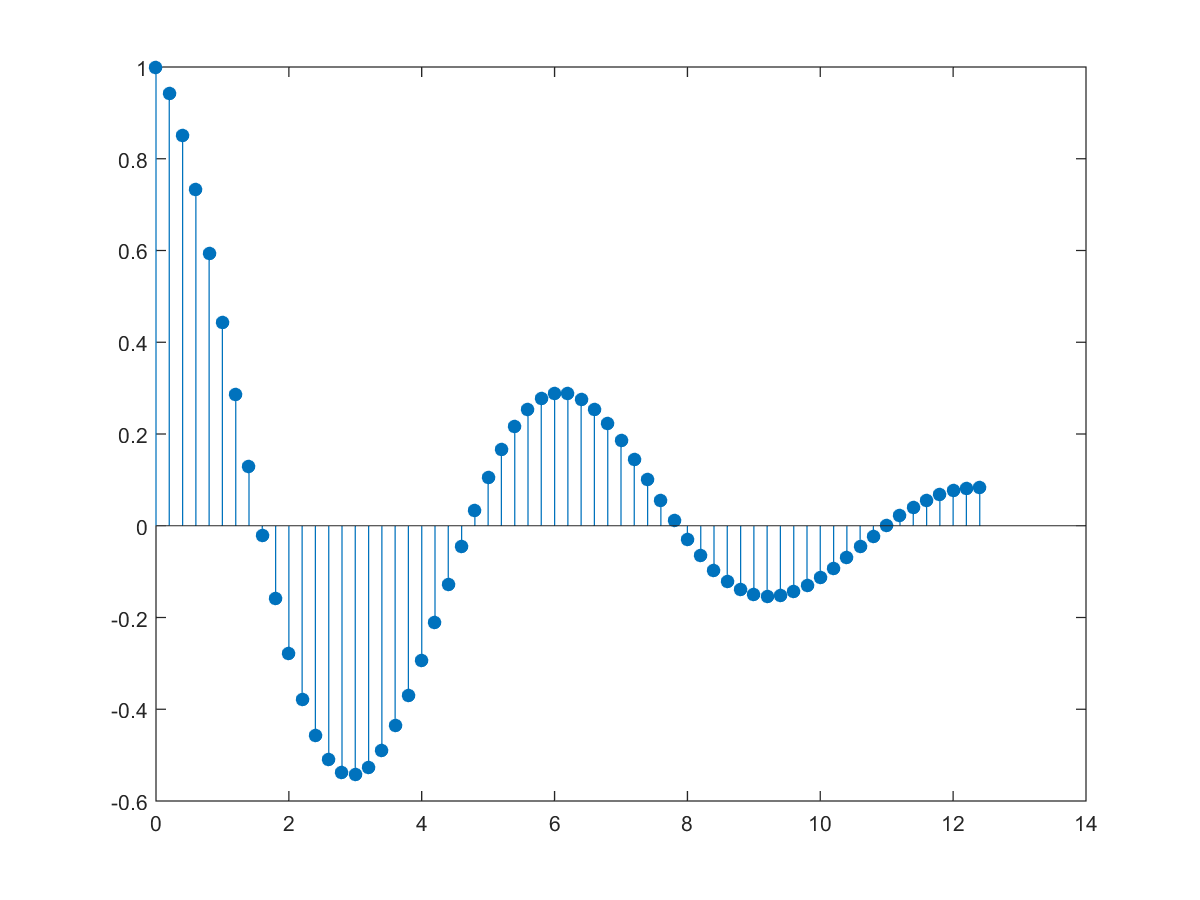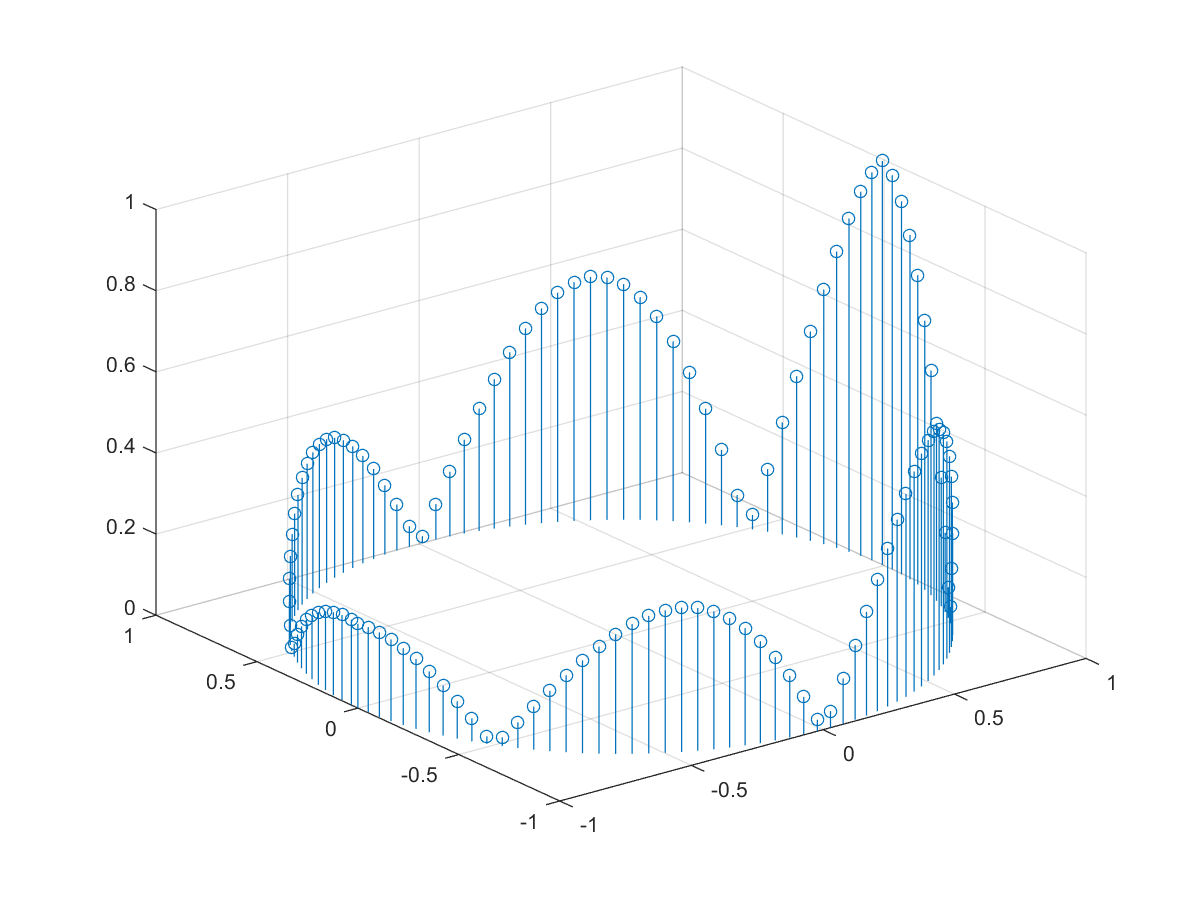5-4 嚙踝蕭嚙踝蕭嚙踝蕭嚙踝蕭嚙?

Example 1: 05-特殊圖形/stem01.mt = 0:0.2:4*pi; y = cos(t).*exp(-t/5); stem(t, y)Example 2: 05-特殊圖形/stem02.mt = 0:0.2:4*pi; y = cos(t).*exp(-t/5); stem(t, y, 'fill');Example 3: 05-特殊圖形/stem301.mtheta = -pi:0.05:pi; x = cos(theta); y = sin(theta); z = abs(cos(3*theta)).*exp(-abs(theta/2)); stem3(x, y, z);MATLAB程式設計：入門篇